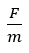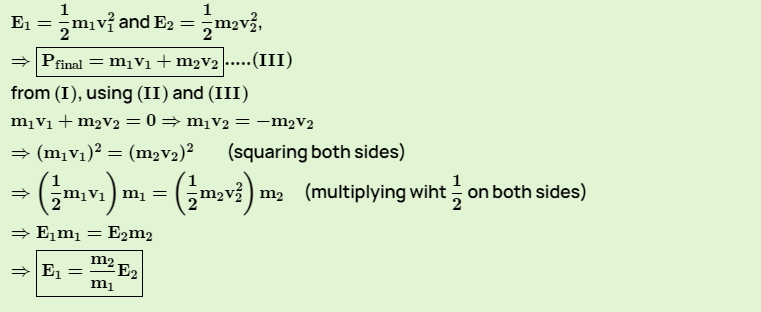Kinetic EnergyThe energy possessed by a body by virtue of it’s motion is called kinetic energy. A body moving with higher speed will possess more kinetic energy than body moving with lower speed.

To derive the expression for the kinetic energy, consider a body having mass m is lying on a smooth horizontal surface . let a constant force (F) displaces the body in it’s own direction though a displacement S. Let the body is at rest initially (u=0) and it’s velocity becomes(v) after travelling the distance (S). Then, the work done (W) by force is,

W=FS.cosϴ    ……(1)

Here, force and the displacement are in same direction, so , ϴ=0 .

W=F.Scos0=F.S   …….(2)

From Newton’s second law of motion, F=ma

or,   a=……..(3)

Using, v2-u2=2aS

or,  v2 – 0 = 2S  [Using equation(3)]

or,   F.S =(1/2)mv2      …….(4)

From equations (2) and (4), we get

W= (½). mv2      ……..(5)

This workdone (W) becomes the kinetic energy (K.E) of the body after displacement(S)

K.E=(1/2)mv2

Therefore, the kinetic energy of a body is equal to one half the product of the mass of the body and square of it’s speed.

Explosion

Further, suppose a stationary mass suddenly explodes into two fragments. Let the masses after explosion be m1,m2 speed v1 , v2 momentum P1 , P2  kinetic energy E1 , E2 .This shows that the kinetic energy due to explosion force is inversely proportional to the mass of the fragment.

#Therefore, if a stationary mass suddenly explodes into two fragments-one heavy and another light, then light fragment have greater kinetic energy than that of heavy fragment. This is the reason that a bullet has greater kinetic energy than the kinetic energy of recoil of gun in case of firing.